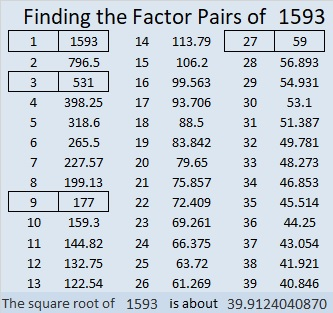# Sorting the Factors of 1593

### Curious Sort of Factors:

1593 has eight factors. Three-fourths of those factors can be made using some or all of its digits. The other two factors seem to be talking about each other. 27 says 177 has two sevens, and 177 says that 27 has one seven. I’ve sorted them into two categories:### Today’s Puzzle:

Can 1593 be expressed using its four digits exactly once and (), +, -, ×, ÷?
Almost, but not quite:
1593 = 3³ × 59¹. We are not allowed to use the 3 twice.
1593 = 531√9. We can use digits as exponents, but we are not allowed to use the square root symbol.
1593 is not a Friedman number because 1593 = 1593 is the only way we can express it using (), +, -, ×, ÷ and only its own digits as numbers or exponents.

Now try this: Can you express each of 1593’s eight factors using all four of its digits exactly once and only (), +, -, ×, ÷? I’ve done a few of them to get you started. (One of them can’t be done, and there is more than one possibility for some of them.)

1 = 1⁵⁹³
3 =
9 = 3(9-5-1)
27 =
59 =
177 =
531 =
1593 = 1593. That’s all we can do for that one.

### Factors of 1593:

• 1593 is a composite number.
• Prime factorization: 1593 = 3 × 3 × 3 × 59, which can be written 1593 = 3³ × 59.
• 1593 has at least one exponent greater than 1 in its prime factorization so √1593 can be simplified. Taking the factor pair from the factor pair table below with the largest square number factor, we get √1593 = (√9)(√177) = 3√177.
• The exponents in the prime factorization are 3 and 1. Adding one to each exponent and multiplying we get (3 + 1)(1 + 1) = 4 × 2 = 8. Therefore 1593 has exactly 8 factors.
• The factors of 1593 are outlined with their factor pairs in the graphic below.### More about the Number 1593:

Consecutive numbers 1592 and 1593 each have eight factors because they are each a cube times a prime.

1593 is the difference of two squares four different ways:
797² – 796² = 1593,
267² – 264² = 1593,
93² – 84² = 1593, and
43² – 16² = 1593.